# Scanner - Cost of Production(2008-2015) Notes | Study Economics Class 11 - Commerce

## Commerce: Scanner - Cost of Production(2008-2015) Notes | Study Economics Class 11 - Commerce

The document Scanner - Cost of Production(2008-2015) Notes | Study Economics Class 11 - Commerce is a part of the Commerce Course Economics Class 11.
All you need of Commerce at this link: Commerce

N. .C. E. R. T Questions

(Q1) Classify following into fixed and variable cost

(a) Rent for shed           (b) Minimum telephone bill
(c) Cost of raw material (d) Wages to permanent staff
(e) Interest on capital    (f) Payment for transportation of goods
(g) Telephone charges beyond the minimum     (h) Daily Wages.
(i) Expenditure on raw material (j) Excise duty and Sales Tax

(Q2) How does TFC changes when output changes

(Q3) How is total variable cost derived from marginal cost schedule ?

(Q4) What is the general shape of AFC, MC , AC curves?

(Q5) What will happen to ATC when MC > ATC ?

(Q6) Distinguish between fixed and variable cost ?

(Q7) With the help of a suitable diagram , explain the relationship between TC, TFC, and TVC

(Q8) DO ATC and AVC curves intersect? Give reason

(Q9) Why is the MC curve in the short run U - shaped ?

(Q10) Explain the relationship between ATC, AVC and MC with a suitable illustration.

(Q11) During market period all cost are fixed cost . How ?

C. B. S. E. Questions

(Q1) Meaning of Marginal Cost, Fixed Cost / supplementary cost, variable cost ?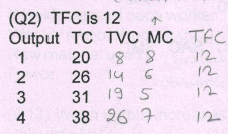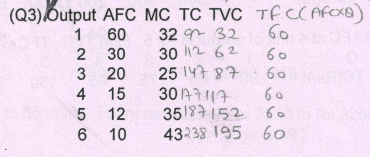(Q4) Why does average fixed cost falls as output rises ?

(Q5) Distinguish between fixed and variable costs ? Give two example of each ?

(Q6) Explain the relationship between MC and AC with the help of cost schedule ?

CBSE 2008 + Sample Paper Questions

(Q1) Distinguish between (i) fixed cost and variable cost giving examples and (ii) average cost and marginal cost giving an example. (4M)

(Q2) A firm’s Average Fixed Cost of producing 2 units of a good is Rs. 9 and given below is its total cost schedule. Calculate its Average Variable Cost and Marginal Cost for each of the given level of output :  (3M)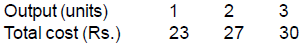(Q3) Define opportunity cost.(1M)

(Q4) Define cost.(1M)

(Q5) Draw average total cost, average variable cost, and marginal cost curves in a single diagram. Also explain the relationship between ATC and AVC.(6M)

(Q6) Explain the relation between (i) ATC and AVC and (ii) MC and AVC.(6M)

CBSE 2009

(Q1) Why is average total cost greater than average variable cost ?(1M)

(Q2) Define fixed costs.(1M)

(Q3) Define marginal cost.(1M)

(Q4) Complete the following table :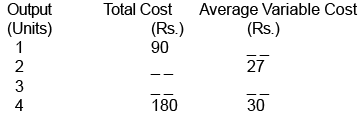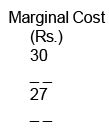(Q5) Complete the following table :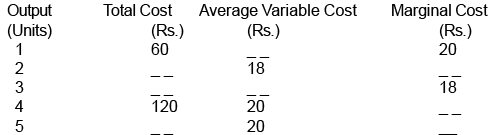(Q6) Complete the following table :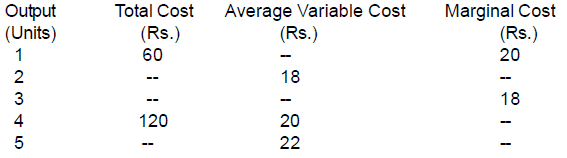(Q7) Given below is cost schedule of a firm . Its AFC is 20 rupees when it produces 3 units.Calculate MC and ATC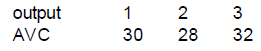(Q8) State true or false with reason   (6M)

(i) As output is increased, the difference between average total cost and average variable falls and utimately becomes zero
(ii) Average cost falls only when marginal cost falls.
(iii) The difference between average total cost and average variable cost is constant.

(Q9) State true or false with reason (a) AVC can fall when MC is rising (b) The difference between TC and TVC fall with increase in output (CBSE {D})

CBSE 2010 + Sample Paper Questions

(Q1) State true or false. Give reasons for your answer : As soon as marginal cost starts rising,average variable cost also starts rising.(2M)

(Q2) A firm’s average fixed cost, when it produces 2 units, is Rs. 30. Its average total cost schedule is given below. Calculate its marginal cost and average variable cost at each level of  output. (3M)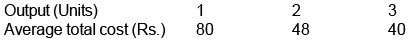(Q3) Given below is the cost shedule of a firm. Its total fixed cost is Rs. 120. Calculate of a firm. Calculate the marginal cost and average variable cost at each level of output.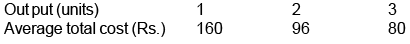(Q4) From the following cost schedule of a firm, calculate marginal cost and average variable cost at each level of output.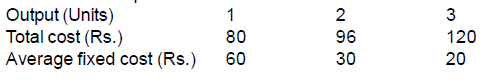(Q5) Give the meaning of marginal cost.(1 M)

(Q6) What is meant by cost in economics ?(1 M)

(Q7) State the distinction between explicit cost and implicit cost. Give an example of each.(3 M)

(Q8) Why does the difference between Average Total Cost and Average Variable Cost decrease with an increase in the level of output ? Can these two be equal at some level of output

CBSE 2011 & CBSE 2012

(Q1) Distinguish between explicit cost and implicit cost and give examples.(3 marks)

(Q2) Define marginal cost. Explain its relation with average cost.(4 marks)

OR
Define variable cost. Explain the behaviour of total variable cost as output increases.(4 marks)

(Q3) Distinguish between explicit cost and implicit cost and give examples.(3 marks)

(Q4) An individual is both the owner and manager of a shop taken on rent . Identify implicit and explicit costs from this information . Explain  CBSE (D) 2012

(Q5) A producer borrows money and opens a shop . The shop premises is owned by him . Identify Identify implicit and explicit costs from this information . Explain CBSE (D) 2012

(Q6) A producer starts a business by investing his own saving . He employs a manager to look after it .Identify implicit and explicit costs from this information . ExplainCBSE (D) 2012

(Q7) What is the behaviour of Total Variable Cost, as output increases? (1 mark)

(Q8) A farmer takes a farm on rent and carries on farming with the help of family members. Identify explicit and implicit costs from this information. Explain.(3 marks)

(Q9) Draw Total Variable Cost, Total Cost, and Total Fixed Cost curves in a single diagram. or Explain the behaviour of total cost as output increases. (3 marks)

(Q10) A producer starts a business by investing his own savings and hiring the labour. Identify implicit costs from this information. Explain. (3 marks)

(Q11) What is Opportunity Cost ? Explain with the help of an example.  (3 marks)

(Q12) A producer borrows money and starts a business. He himself looks after the business. Identify implicit and explicit costs from this information. Explain. (3 marks)

CBSE 2013

(Q1) Define Marginal Cost

Ans: Addition to total cost on producing one more unit.

(Q2) Give two examples of fixed costs

Ans:  Rent , Salary of permanent employee, etc. (any two) ½×2

(Q3 ) Give two examples of variable  costs

Ans: Expenditure on raw materials, casual labour, etc (any two)

(Q4) State the behavior of Total Variable Cost. Draw Total Variable Cost, Total Cost and Total Fixed Cost curves in a single diagram. (4 marks)

(Q5) Show with the help of numerical example that AC is constant when MC is equal to it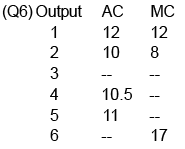Sample Paper 2014-15 & 15-16 & 16-17

(Q1) If it is given that the total variable cost for producing 15 units of output is Rs. 3000 and for 16 units is Rs. 3,500. Find the value of Marginal Cost.

Ans: 500

(Q2) If a firm’s production department data says that the total variable cost for producing 8 units and 10 units of output is 2,500 and 3,000 respectively, marginal cost of 10th unit will be

a. 100 b. 150 c. 500 d. 250

Ans:  (d) 250

(Q3) The total cost at 5 units of output is Rs 30. The fixed cost is Rs 5. The average variable cost at 5 units of output is :

(a) Rs 25 (b) Rs 6 (c) Rs 5 (d)  Re 1

Ans: (c)

(Q4) State true or false

(a)  AFC curve is a rectangular hyperbola curve.

(b)  Total cost rises only when marginal cost rises

(c) With increase in level of output, average fixed cost goes on falling till it reaches zero.

Ans:

(a) True : Since TFC remains unchanged / constant.

(b) False : Total cost rises at a diminishing rate when marginal cost falls and total cost rises at an increasing rate when marginal cost increases.

(c) False :  TFC is constant and positive. So with an increase in output AFC will fall but can never be zero.

(Q5) Justify the statement, ‘In economics, normal profits are always a part of total cost’. (3)

Ans: The given statement is correct .

Normal profit is defined as the minimum reward that is just sufficient to keep the entrepreneur supplying his factor service  Since total cost includes payment made to primary inputs: land, labour, capital andenterprise, total cost includes rent, wages, interest and (normal) profits

(Q6) Find out (a) explicit cost and (b) implicit cost from the following :(4 marks)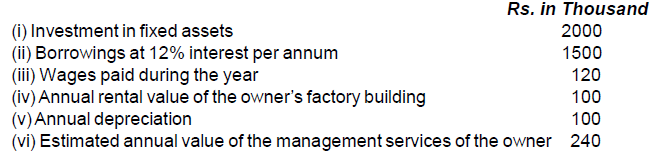Ans. 400, 400

C.B.S.E Paper 2014

(Q1) Define variable cost.  (1 mark)

Ans: The cost which changes with change in output is called variable cost.

(Q2) What is cost in economics ?  (1 mark)

Ans: Cost in economics refers to the sum of actual money expenditure on inputs and the estimated value of the free inputs supplied by the owner.

(Q3) Draw a total variable cost curve and a total cost curve in a single diagram.(3M)

(Q4) Explain behaviour of Average Fixed Cost (AFC). Use diagram.(3M)

Ans: AFC falls continuously as output is increased.It is because, even when output is increased TFC remains unchanged.

(Q5) Explain the relation between total Cost and Marginal cost

Ans: W hen TC rises at a decreasing rate, MC falls. When TC rises at an increasing rate MC rises. When TC rises at a constant rate MC is constant

Sample Paper 2015

(Q1) The total cost at 5 units of output is Rs. 30. The fixed cost is Rs. 5. The average variable cost at 5 units of output is :(1 M)

(a) Rs. 25 (b) Rs. 6 (c) Rs. 5 (d) Rs.1

(a) Total cost rises only when marginal cost rises.

C.B.S.E Paper 2015

(Q1) What is the behaviour of (a) Average Fixed Cost and (b) Average Variable Cost as more and more units of a good are produced ?(4 M)

Ans:  (a) AFC falls continuously as more and more output is produced. (2M)

(b) AVC falls initially and after a level of output, starts rising as more and more output is produced. (2M)

(Q2) The average fixed cost at 4 units of output is Rs.20. Average variable cost at 5 units of output is Rs.40. Average cost of producing 5 units is :[AI (C)]

(a) Rs.20 (b) Rs.40 (c) Rs. 56 (d) Rs. 60

(Q3) Giving reasons, state whether the following statements are true or false?[AI (C)]

(a) The difference between AC and AVC is always constant. (b) When MC rises, AVC must rise. (c) Average cost will rise only when marginal cost rises.

(Q4) State the relationship between :[AI (C)]

(a) Marginal cost and average variable cost (b) Total cost and marginal costExplain the

(Q5) Define cost. State the relation between marginal cost and average variable cost.(1M)

Ans: Cost in economics refers to the sum of actual money expenditure on inputs and the imputed expenditure in the form of inputs supplied by the owners including normal profit.

If MC < AVC , then AVC falls

If MC = AVC, then AVC is constant

If MC > AVC, then AVC rises                      (Diagram not required)

(Q6) The average fixed cost at 4 units of output is Rs 20. Average variable cost at 5 units of output is Rs 40. Average cost of producing 5 units is rupees  : (1)

(a)  20 (b) 40 (c)  56 (d)  60

Ans: (c)

(Q7)Giving reasons, state whether the following statements are true or false : The difference between average cost and average variable cost is always constant

Ans: False, The difference between AC and AVC is due to AFC. As output is increased AFC falls, so the difference between AC and AVC falls.

The document Scanner - Cost of Production(2008-2015) Notes | Study Economics Class 11 - Commerce is a part of the Commerce Course Economics Class 11.
All you need of Commerce at this link: CommerceUse Code STAYHOME200 and get INR 200 additional OFF

## Economics Class 11

201 videos|195 docs|64 tests

Track your progress, build streaks, highlight & save important lessons and more!

,

,

,

,

,

,

,

,

,

,

,

,

,

,

,

,

,

,

,

,

,

;